Open in App
Not now

# How to Create a Nested For Loop in R?

• Last Updated : 02 Feb, 2022

A loop in a programming language is a sequence of instructions executed one after the other unless a final condition is met. Using loops is quite frequent in a program.

## Need of a loop

Let us consider a scenario where we want to print natural numbers from 1 to 3. We can simply print them one by one. But suppose the range is high, 1 to 100 or 1 to 10000 or even 1 to 100000, then printing them one by one is a tedious task. In such cases, loops are quite efficient and also improve the readability of the source code. In R, loops are broadly classified into three categories:  for, while, and repeat. This article focuses upon the working of For-loop in R.

## For loop in R:

For loop is one of the commonly used types of loops. It is a control statement that is used in scenarios where multiple statements are required to be executed. In R, for loop follows the below syntax.

Syntax:

for (element in sequence) {

// body

}

Here, an element is the actual value in the sequence one after the another and starting from the beginning.

## Nested for loop in R:

A nested for-loop has a for-loop inside of another for-loop. For each of the iteration in the outer for-loop, the inner loop will be executed unless a final condition is met for the inner loop. Once an inner for-loop is executed for a particular outer iteration then the outer for-loop goes for the next iteration and now the inner loop will be executed for this iteration. This process repeats itself till the final condition is met for the outer for-loop. Nested for-loop can be visualized as iterating over integer coordinates one by one in a two-dimensional space. For example, printing

```(0,0)   (0,1)   (0,2)
(1,0)   (1,1)   (1,2)
(2,0)   (2,1)   (2,2)
(3,0)   (3,1)   (3,2) ```

Below is the syntax of nested for-loop in R.

Syntax:

for (element1 in sequence1) {

for(element2 in sequence2)

// body

}

Example 1: In the below program we are iterating over 0 to 3 in the outer loop and for each value in the outer for-loop, we are iterating over 0 to 3 in the inner for-loop.

## R

 `# R program to illustrate the working of``# nested for-loop `` ` `# Iterating over 0 to 3 in the outer``# for-loop``for ``(x ``in` `0:3){``       ` `      ``# Iterating over 0 to 3 in the inner ``    ``# for-loop``      ``for ``(y ``in` `0:3)``    ``{``        ``# Print x and y ``          ``print``(``paste``(``"("``, x, ``","``, y, ``")"``))`` ` `    ``}``}`

Output: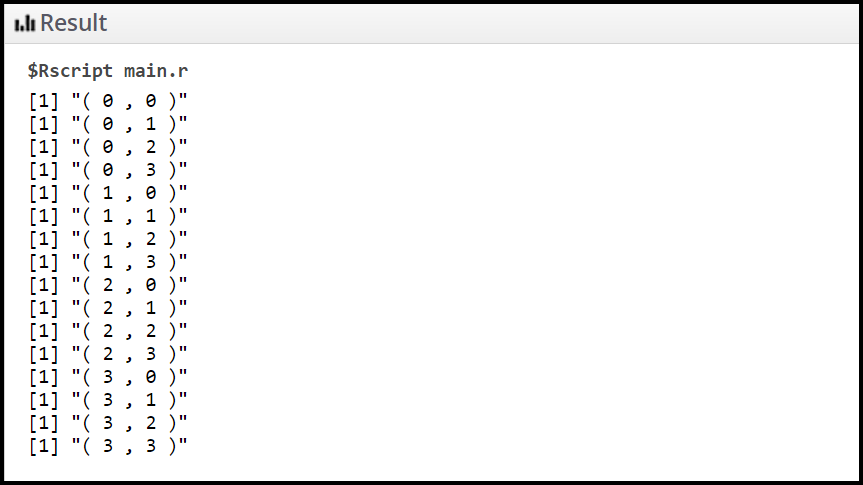Example 2: In the below program we are iterating over 1 to 5 in the outer loop and for each of the values in the outer for-loop, we are iterating over 1 to 5 in the inner for-loop and simultaneously printing the sum of the two values.

## R

 `# R program to illustrate the working ``# of nested for-loop`` ` `# Iterating over 1 to 5 in the outer ``# for-loop``for``(number1 ``in` `1:5)``{``      ``# Iterating over 1 to 5 in the inner ``    ``# for-loop``    ``for``(number2 ``in` `1:5)``    ``{``        ``# Print the sum of number1 and number2``        ``print``(``paste``(number1, ``"+"``, number2, ``"="``, ``                    ``number1 + number2));``    ``}``}`

Output: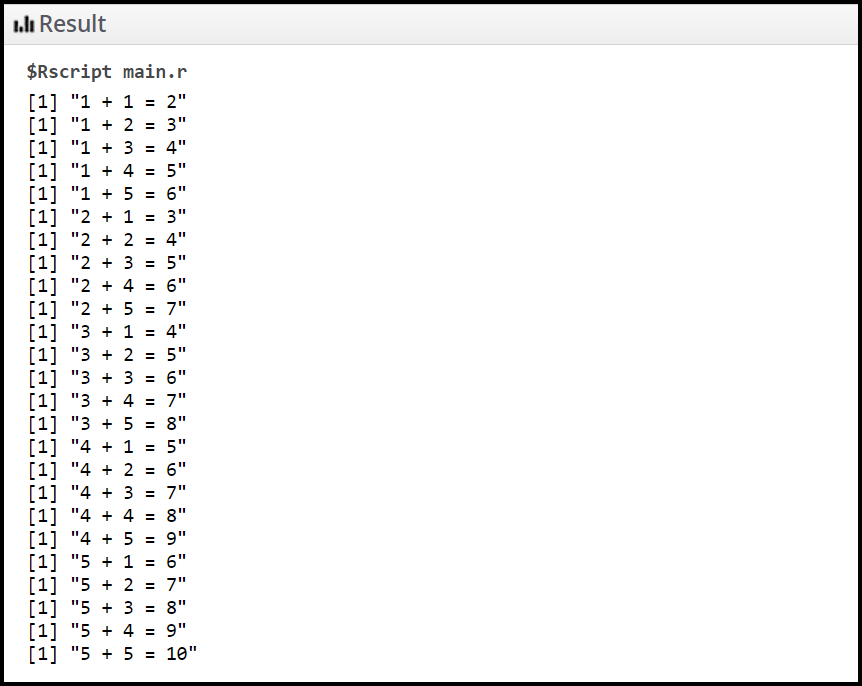## Next statement:

Most of the languages provide functionality using which we can skip the current iteration at the moment and go for the next iteration if it exists. For this purpose, we have the “next” statement in R.

Example 1: In the below program we are skipping the inner for-loop if the value of number1 is equal to one.

## R

 `# R program to illustrate the working``# of next statement in nested for-loop`` ` `# Iterating over outer loop``for``(number1 ``in` `1:5)``{``      ``if``(number1 == 1)``      ``next``      ``# Iterating over outer loop``    ``for``(number2 ``in` `1:5)``    ``{``        ``# Print the sum of number1 and number2``        ``print``(``paste``(number1, ``"+"``, number2, ``"="``,``                    ``number1 + number2));``    ``}``}`

Output: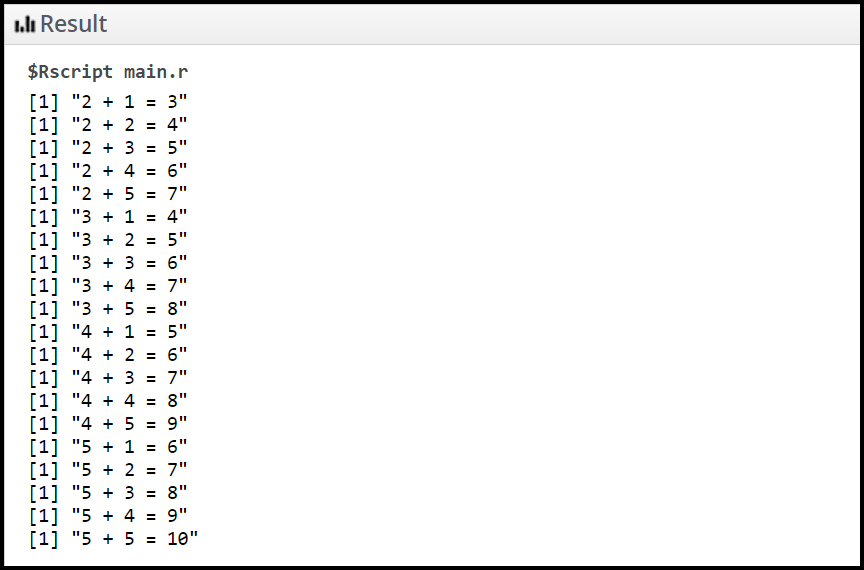Example 2: In the below program we are skipping the print statement if the value of number2 is equal to one in the inner for-loop.

## R

 `# R program to illustrate the working``# of next statement in nested for-loop`` ` `# Iterating over outer loop``for``(number1 ``in` `1:5)``{``      ``# Iterating over outer loop``    ``for``(number2 ``in` `1:5)``    ``{``        ``# If number1 is equal to 1``        ``# Then skip the print statement below``        ``# and move to the next iteration``        ``if``(number2 == 1)``              ``next``       ` `        ``# Print the sum of number1 and number2``        ``print``(``paste``(number1, ``"+"``, number2, ``"="``,``                    ``number1 + number2));``    ``}``} `

Output: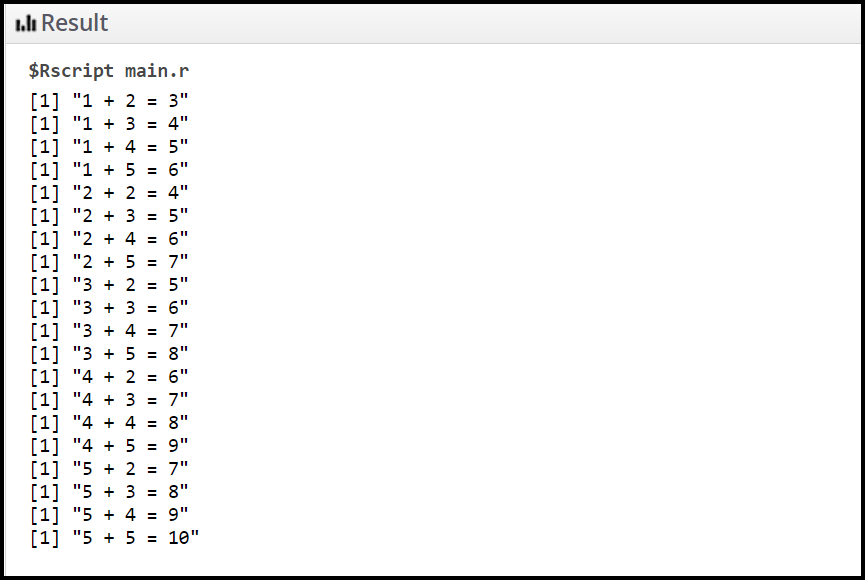## Break statement:

Most of the languages provide functionality using which we can stop the iteration of for-loop at the moment and come out of the current for-loop scope. For this purpose, we have the “break” statement in R. Break statement in R is the same as the break statement in C or C++.

Example 1: In the below program the flow of control of the outer for-loop comes out of the scope once the value of number1 reaches towards 2.

## R

 `# R program to illustrate the working``# of break statement in nested for-loop`` ` `# Iterating over 1 to 5 in outer for-loop``for``(number1 ``in` `1:5)``{``    ``# If number1 is equal to 2``    ``# then break the flow of control``    ``# and come out of the outer loop``      ``if``(number1 == 2)``      ``break``       ` `    ``# Iterating over 1 to 5 in inner for-loop``    ``for``(number2 ``in` `1:5)``    ``{``        ``# Print the sum of number1 and number2``        ``print``(``paste``(number1, ``"+"``, number2, ``"="``, ``                    ``number1 + number2));``    ``}``}`

Output: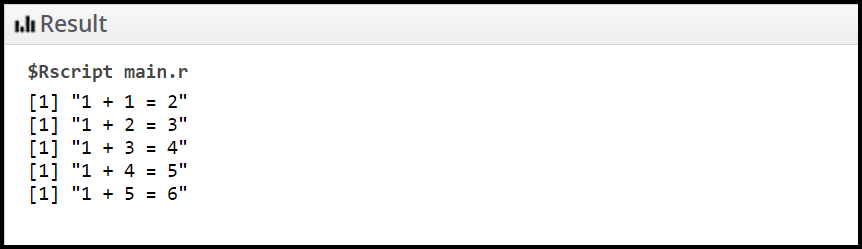Example 2: In the below program the flow of control of the inner for-loop comes out of the scope once the value of number2 reaches towards 2.

## R

 `# R program to illustrate the working of``# break statement in nested for-loop`` ` `# Iterating over 1 to 5 in the outer for-loop``for``(number1 ``in` `1:5)``{`` ` `# Iterating over 1 to 5 in the inner for-loop``    ``for``(number2 ``in` `1:5)``    ``{``        ``# If number2 is equal to 2 then stop``        ``# the flow of control``        ``# and come out of the inner for-loop at the moment``        ``if``(number2 == 2)``            ``break``             ` `          ``# Print the sum of number1 and number2``        ``print``(``paste``(number1, ``"+"``, number2, ``"="``, ``                    ``number1 + number2));``    ``}``}`

Output: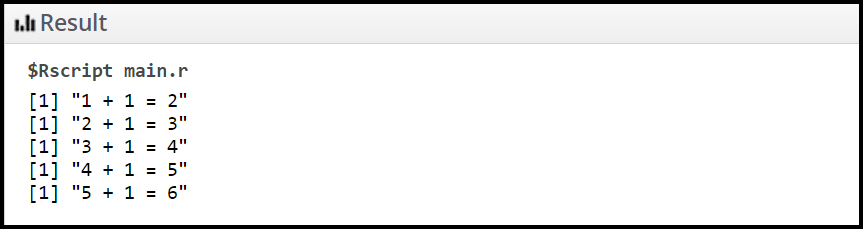My Personal Notes arrow_drop_up